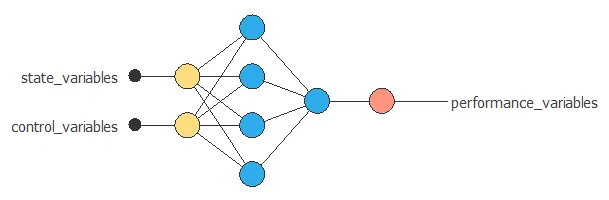﻿ Process optimization using machine learning

# Process optimization using machine learningTracking the behavior of a system allows us to predict its behavior and improve its performance.

However, the performance of a process may depend on a large number of variables. Therefore, sophisticated methods and tools are needed to untangle all these factors effectively.

This article explains how to use machine learning and Neural Designer to model engineering systems and optimize industrial processes.

Contents:

## Data set

The data set contains measurements from our system or process.

It comprises state, control, and performance variables.

State variables are those inputs that determine the system's performance and are not actionable by the company's technicians.

Some examples of state variables are:

• The ambient temperature in a combined cycle power plant.
• The water salinity in a desalination plant.

Control variables are those inputs that determine the performance of the system and can be set by the company's technicians.

Two examples of the control variables are:

• The velocity of an aircraft.
• The combustion airflow in a furnace.

Performance variables are the outputs of the system and depend on the state and the control variables.

Performance optimization can consider different targets:### PROCESS EFFICIENCY### ENERGY CONSUMPTION### GAS EMISSIONS## Mathematical model

The model of a process is a mathematical description that adequately predicts the physical system's response to all anticipated inputs.

More specifically, it relates the performance variables with the state and control variables.

$$performance\_variables = function(state\_variables, control\_variables)$$

Neural networks are algorithms used to fit multi-dimensional and non-linear functions from data sets.The inputs to the neural network include the state and control variables. The outputs from the neural network are the predicted performance variables of the system for that scenario.

## Response optimization

The objective of the response optimization algorithm is to exploit the mathematical model to look for optimal operating conditions.

Indeed, the predictive model allows us to simulate different operating scenarios and adjust the control variables to improve efficiency.

More specifically, we can formulate a performance optimization task as follows:

For a given set of states, determine the controls that minimize or maximize the performance variables.

The next figure illustrates the response optimization process.As we can see, for a given state value, s, the control value, c*, minimizes the performance value.

A performance optimization problem might also include a set of constraints on the system's inputs and outputs.

An example is to minimize the fuel consumption of an aircraft while maintaining the speed at the desired value.

## Conclusions

Machine learning is a powerful technique to predict the performance of engineering systems.

That allows us to simulate different operating scenarios and adjust the control parameters to improve efficiency.

Some examples of performance optimization are improving process efficiency or reducing energy consumption.

Neural Designer uses neural networks to model the behavior of systems. It also contains response optimization algorithms to fine-tune the control variables and optimize performance.